Updating search results...

# 5 Results

View
Selected filters:
• mathematician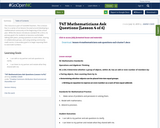Conditional Remix & Share Permitted
CC BY-NC-SA
Rating
0.0 stars

This resource is part of Tools4NCTeachers.

This is lesson four in a series of six lessons focused around developing a mathematical community at the beginning of the school year. While this lesson introduces standard NC.2.OA.3, its primary goal is for students to become comfortable talking with peers, posing questions to each other, sharing in collaborative groups, and using existing community math norms. A secondary goal is to begin exposing them to even/odd numbers.

Subject:
Mathematics
Material Type:
Lesson
Lesson Plan
11/06/2019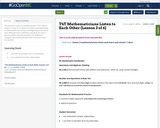Conditional Remix & Share Permitted
CC BY-NC-SA
Rating
0.0 stars

This resource is part of Tools4NCTeachers.

This is lesson three in a series of six lessons focused around developing a mathematical community at the beginning of the school year. While this lesson addresses standards NC.2.OA.2 and NC.2.NBT.4, the primary goal is for students to recognize that mathematicians listen to and learn from one another.

Subject:
Mathematics
Material Type:
Lesson
Lesson Plan
11/06/2019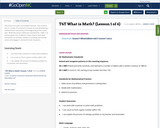Conditional Remix & Share Permitted
CC BY-NC-SA
Rating
0.0 stars

This resource is part of Tools4NCTeachers.

This is lesson one in a series of six lessons focused around developing a mathematical community at the beginning of the school year. While this lesson addresses standard NC.1.NBT.7, its primary goal is for students to learn how to work with classmates on activities related to counting and number sense and engage in real-world math situations.

Subject:
Mathematics
Material Type:
Lesson
Lesson Plan
11/06/2019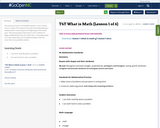Conditional Remix & Share Permitted
CC BY-NC-SA
Rating
0.0 stars

This resource is part of Tools4NCTeachers.

This is lesson one in a series of six lessons focused around developing a mathematical community at the beginning of the school year. The primary goal of this lesson is for students to begin establishing norms for math class. A secondary goal is to start exploring a non-standard problem that has more than one possible solution.

Subject:
Mathematics
Material Type:
Lesson
Lesson Plan
11/06/2019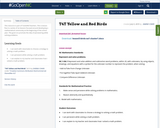Conditional Remix & Share Permitted
CC BY-NC-SA
Rating
0.0 stars

This resource is part of Tools4NCTeachers.

This is lesson five in a series of six lessons focused around developing a mathematical community at the beginning of the school year. The goal is to promote the idea of persevering while solving problems.

Subject:
Mathematics
Material Type:
Lesson
Lesson Plan